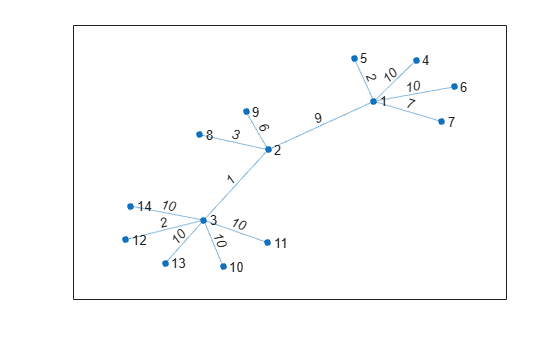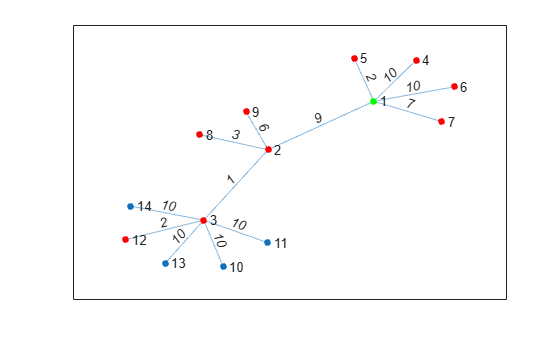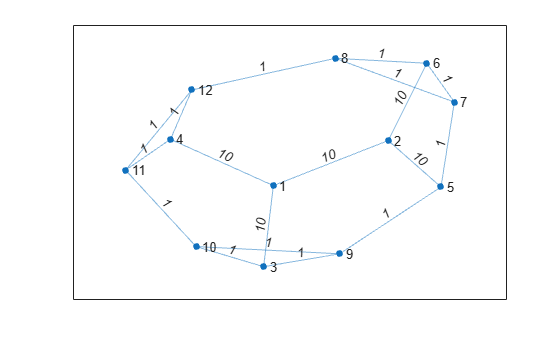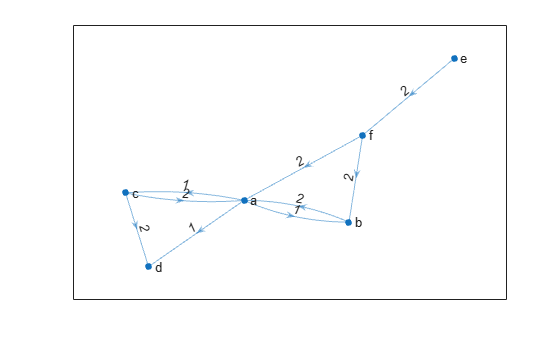Documentation

### This is machine translation

Mouseover text to see original. Click the button below to return to the English version of the page.

# nearest

## Syntax

``nodeIDs = nearest(G,s,d)``
``nodeIDs = nearest(G,s,d,Name,Value)``
``````[nodeIDs,dist] = nearest(___)``````

## Description

example

````nodeIDs = nearest(G,s,d)` returns all nodes in graph `G` that are within distance `d` from node `s`. If the graph is weighted (that is, if `G.Edges` contains a variable `Weight`), then those weights are used as the distances along the edges in the graph. Otherwise, all edge distances are taken to be `1`.```

example

````nodeIDs = nearest(G,s,d,Name,Value)` uses additional options specified by one or more name-value pair arguments. For example, if `G` is a weighted graph, then `nearest(G,s,d,'Method','unweighted')` ignores the edge weights in graph `G` and instead treats all edge weights as `1`.```

example

``````[nodeIDs,dist] = nearest(___)``` additionally returns the distance to each of the nearest neighbors, such that `dist(j)` is the distance from source node `s` to the node `nodeIDs(j)`. You can use any of the input argument combinations in previous syntaxes.```

## Examples

collapse all

Create and plot a graph with weighted edges.

```s = [1 1 1 1 1 2 2 2 3 3 3 3 3]; t = [2 4 5 6 7 3 8 9 10 11 12 13 14]; weights = randi([1 10],1,13); G = graph(s,t,weights); p = plot(G,'Layout','force','EdgeLabel',G.Edges.Weight);```Determine which nodes are within a radius of 15 from node 1.

`nn = nearest(G,1,15)`
```nn = 9×1 5 7 2 3 4 6 8 12 9 ```

Highlight the source node as green and the nearest neighbors as red.

```highlight(p,1,'NodeColor','g') highlight(p,nn,'NodeColor','r')```Create and plot a graph with weighted edges.

```s = [1 1 1 2 2 6 6 7 7 3 3 9 9 4 4 11 11 8]; t = [2 3 4 5 6 7 8 5 8 9 10 5 10 11 12 10 12 12]; weights = [10 10 10 10 10 1 1 1 1 1 1 1 1 1 1 1 1 1]; G = graph(s,t,weights); plot(G,'EdgeLabel',G.Edges.Weight)```Determine which nodes are within a radius of 5 from node 3, and return the distance to each node.

`[nn,dist] = nearest(G,3,5)`
```nn = 9×1 9 10 5 11 4 7 12 6 8 ```
```dist = 9×1 1 1 2 2 3 3 3 4 4 ```

Create and plot a directed graph with weighted edges.

```s = {'a' 'a' 'a' 'b' 'c' 'c' 'e' 'f' 'f'}; t = {'b' 'c' 'd' 'a' 'a' 'd' 'f' 'a' 'b'}; weights = [1 1 1 2 2 2 2 2 2]; G = digraph(s,t,weights); plot(G,'EdgeLabel',G.Edges.Weight)```Determine the nearest nodes within a radius of 1 from node `'a'`, measured by outgoing path distance from node `'a'`.

`nn_out = nearest(G,'a',1)`
```nn_out = 3x1 cell array {'b'} {'c'} {'d'} ```

Determine all of the nodes that have incoming paths leading to node `'a'` by specifying the radius as `Inf`.

`nn_in = nearest(G,'a',Inf,'Direction','incoming')`
```nn_in = 4x1 cell array {'b'} {'c'} {'f'} {'e'} ```

## Input Arguments

collapse all

Input graph, specified as either a `graph` or `digraph` object. Use `graph` to create an undirected graph or `digraph` to create a directed graph.

Example: `G = graph(1,2)`

Example: `G = digraph([1 2],[2 3])`

Source node, specified as a node index or a node name in one of the forms in this table.

ValueExample
Scalar node index`1`
Character vector node name`'A'`
String scalar node name`"A"`

Example: `nearest(G,3,1)`

Example: `nearest(G,'a',5)`

Neighbor distance radius, specified as a numeric scalar.

Example: `nearest(G,3,1)`

Example: `nearest(G,'a',2.5)`

### Name-Value Pair Arguments

Specify optional comma-separated pairs of `Name,Value` arguments. `Name` is the argument name and `Value` is the corresponding value. `Name` must appear inside quotes. You can specify several name and value pair arguments in any order as `Name1,Value1,...,NameN,ValueN`.

Example: ```[nodeIDs,dist] = nearest(G,s,5,'Method','unweighted','Direction','incoming')```

### Note

The `'Direction'` option can only be specified with directed graphs.

Direction of distance measurement, specified as the comma-separated pair consisting of `'Direction'` and one of the options in this table.

OptionDescription
`'outgoing'` (default)Distances are calculated using paths going out from source node `s`.
`'incoming'`Distances are calculated using paths coming in to source node `s`.

Example: `nearest(G,s,d,'Direction','incoming')`

Shortest path algorithm, specified as the comma-separated pair consisting of `'Method'` and one of the options in this table.

OptionDescription
`'auto'` (default)

The `'auto'` option automatically selects the algorithm:

• `'unweighted'` is used for `graph` and `digraph` inputs with no edge weights.

• `'positive'` is used for all `graph` inputs that have edge weights, and requires the weights to be nonnegative. This option is also used for `digraph` inputs with nonnegative edge weights.

• `'mixed'` is used for `digraph` inputs whose edge weights contain some negative values. The graph cannot have negative cycles.

`'unweighted'`

Breadth-first computation that treats all edge weights as `1`.

`'positive'`

Dijkstra algorithm that requires all edge weights to be nonnegative.

`'mixed'` (only for `digraph`)

Bellman-Ford algorithm for directed graphs that requires the graph to have no negative cycles.

While `'mixed'` is slower than `'positive'` for the same problem, `'mixed'` is more versatile as it allows some edge weights to be negative.

### Note

For most graphs, `'unweighted'` is the fastest algorithm, followed by `'positive'` and `'mixed'`.

Example: `nearest(G,s,d,'Method','positive')`

## Output Arguments

collapse all

Nearest neighbor node IDs, returned as node indices if `s` is numeric, or as node names if `s` is a node name. The nodes are sorted from nearest to furthest. `nodeIDs` is empty if no nodes are within the specified distance. `nodeIDs` never contains the source node `s` even if the graph has self-loops.

Use `H = subgraph(G,[s; nodeIDs])` to extract a subgraph of the nearest neighbors from the original graph `G`.

Neighbor distances, returned as a vector. `dist(j)` is the distance from source node `s` to neighboring node `nodeIDs(j)`.